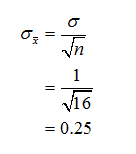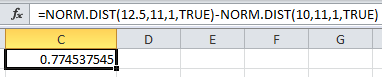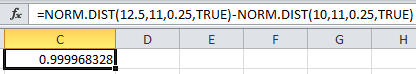# Suppose the age that children learn to walk is normally distributed with mean 11 months and standard deviation 1 month. 16 randomly selected people were asked what age they learned to walk. Round all answers to 4 decimal places where possible.What is the distribution of XX? XX ~ N(,)What is the distribution of ¯xx¯? ¯xx¯ ~ N(,)What is the probability that one randomly selected person learned to walk when the person was between 10 and 12.5 months old? For the 16 people, find the probability that the average age that they learned to walk is between 10 and 12.5 months old. For part d), is the assumption that the distribution is normal necessary? YesNoFind the IQR for the average first time walking age for groups of 16 people.Q1 =  monthsQ3 =  monthsIQR:  months

Question
82 views

Suppose the age that children learn to walk is normally distributed with mean 11 months and standard deviation 1 month. 16 randomly selected people were asked what age they learned to walk. Round all answers to 4 decimal places where possible.

1. What is the distribution of XX? XX ~ N(,)
2. What is the distribution of ¯xx¯? ¯xx¯ ~ N(,)
3. What is the probability that one randomly selected person learned to walk when the person was between 10 and 12.5 months old?
4. For the 16 people, find the probability that the average age that they learned to walk is between 10 and 12.5 months old.
5. For part d), is the assumption that the distribution is normal necessary? YesNo
6. Find the IQR for the average first time walking age for groups of 16 people.
Q1 =  months
Q3 =  months
IQR:  months
check_circle

Step 1

Let X be the age that children learn to walk.

It is given that X is normally distributed with mean 11 months and standard deviation is 1 month.

1).

The distribution of X is given as, X~N(µ=11, σ=1).

2).

Here, the sample size, n is 16.

It is known that the mean of the sampling distribution is also the sample mean.

The standard deviation of the sampling distribution is given as follows:Hence, the sampling distribution of mean age that children learn to walk is given as, X-bar ~ N(µ=11, σ=0.25).

Step 2

3).

Probability that the randomly selected person learned to walk between 10 and 12.5 months can be obtained by entering the formula “=NORM.DIST(12.5,11,1,TRUE)-NORM.DIST(10,11,1,TRUE)” in EXCEL.4).

Probability that the mean age that they learned to walk is between 10 and 12.5 months can be obtained by entering the formula “=NORM.DIST(12.5,11,0.25,TRUE)-NORM.DIST(10,11,0.25,TRUE)” in EXCEL....

### Want to see the full answer?

See Solution

#### Want to see this answer and more?

Solutions are written by subject experts who are available 24/7. Questions are typically answered within 1 hour.*

See Solution
*Response times may vary by subject and question.
Tagged in